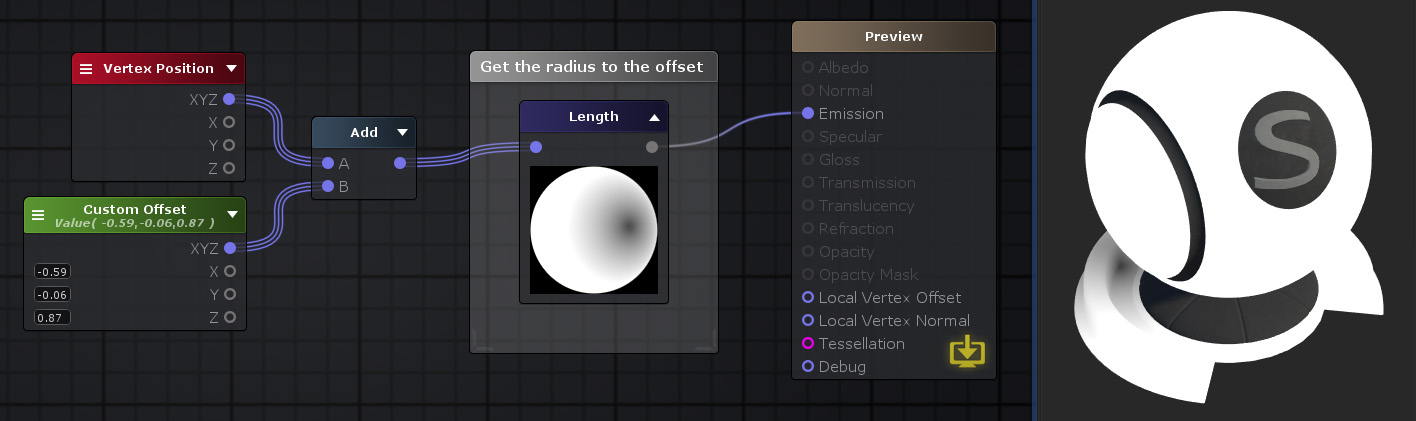## Length Node

The Length node outputs the euclidean length of the Input vector which can be seen as the following operation Sqrt( Dot( Input, Input )).Nodes used: Vertex Position, Vector3, Add, Length

Node Parameter Description Default Value
Input Vector for which the length will be calculated. Only visible if the respective input port is not connected.
Input Port Description Type
Input Vector for which the length will be calculated. Float4 

1. ^ Port automatically adapts to all connection types except Matrices and Sampler types.